# Oblique slab dieletric material in a capacitor

stefano77
Thread moved from the technical forums and user has been reminded to always show their work on schoolwork problems
With reference to picture, l have an oblique slab of material dieletric in a capacitor. The plates of capacitor are infinite and far. How can calculate E and D in dieletric?

#### Attachments

•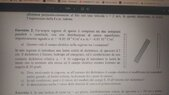physics problem.jpg
51.5 KB · Views: 25

Gordianus
Hint: Do you know about boundary conditions for E and D?

Homework Helper
Gold Member
Also, please provide a complete statement of the problem in English. Thanks.

•berkeman
stefano77
first of all sorry for my bad english.
there is a uniform electric field E created by two plates with superficial charge +/- ## \sigma## .
the plates are infinite and far.
you put inside a dielectric material .
Now i am doubtful.
if the outside field is modified l don't know how to use boundary condition,otherwise l do.
But why shouldn' t outside electric field been modified by the slab of dielectric?this seems the way to solve this problem, that is field no modified.

Last edited:
Homework Helper
Gold Member
To modify the external field, the charge on the plates must be modified. You are told that the plates are very far away. So assume that the external field is not modified by the presence of the dielectric slab and proceed.

stefano77
the charge on the plates aren't modified, but ##E_{total}## =##E_{capacitor}##+##E_{dielectric}## is modified, so that you don't know the direction of ##E_{total}## to apply boundary conditions

Homework Helper
Gold Member
the charge on the plates aren't modified, but ##E_{total}## =##E_{capacitor}##+##E_{dielectric}## is modified, so that you don't know the direction of ##E_{total}## to apply boundary conditions
Still, the electrostatic potential everywhere is a solution to the one-dimensional Laplace equation and has the form
##V_1=A_1x+B_1## in the vacuum
##V_2=A_2x+B_2## in the dielectric
Then ##E_{capacitor}=-\dfrac{\partial V_1}{\partial x}## and ##E_{dielectric} =-\dfrac{\partial V_2}{\partial x}.##
You can find the constants by matching boundary conditions, no?

••Delta2 and berkeman
Homework Helper
Gold Member
It might be helpful to first deduce some important properties of the induced surface charge density of the dielectric slab based on the simple geometry of this system. I think you can neglect "edge effects" of the dielectric slab. In other words, treat the dielectric slab as infinite in size (except for the finite thickness). Let ##\sigma_L## and ##\sigma_R## be the induced surface charge densities on the left and right surfaces of the slab, respectively.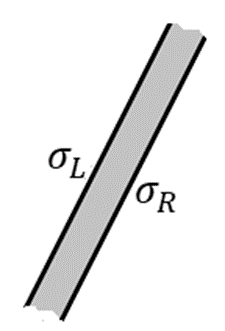What are the signs of ##\sigma_L## and ##\sigma_R##? How do their magnitudes compare?

Can you treat ##\sigma_L## and ##\sigma_R## as uniformly distributed? If so, what is their net contribution to the total electric field outside the dielectric slab?

•Delta2
stefano77
first of all thank you everyone for your answers. it is very kind of you.
then l know ## D_{1n} ## = ## D_{2n}## and ## E_{1t} ## = ## E_{2t}## , but you don't know how is the D and E value ( total) in vacuum.(they are modified by dielectric)
you could apply Laplace equation, but lf l use cartesian Laplace equation in cartesian coordinates it is hard to solve.
you can suppose in vacuum ## E_{total}## is the same as E without dielectric, and l think is the only the say to solve. But why must l suppose that?

Homework Helper
Gold Member
Still, the electrostatic potential everywhere is a solution to the one-dimensional Laplace equation and has the form
##V_1=A_1x+B_1## in the vacuum
##V_2=A_2x+B_2## in the dielectric
Then ##E_{capacitor}=-\dfrac{\partial V_1}{\partial x}## and ##E_{dielectric} =-\dfrac{\partial V_2}{\partial x}.##
You can find the constants by matching boundary conditions, no?
Because the dielectric slab is placed in a not symmetric diagonal way into the capacitor, can we still say that
the potentials will have this simple form?

stefano77
Because the dielectric slab is placed in a not symmetric diagonal way into the capacitor, can we still say that
the potentials will have this simple form?
that's the point. there are not symmetric condition anymore in this case.Then you can't suppose the direction of E vector a priori.

•Delta2
Homework Helper
Gold Member
that's the point. there are not symmetric condition anymore in this case.Then you can't suppose the direction of E vector a priori.
Something tells me that the direction of the surface of the slab relative to the capacitor plates, doesn't matter (otherwise we would have been given an angle of the slab with the horizontal) but let's hear what @kuruman has to say about this..

stefano77
in the text there is an angle with the horizontal ( 30 degrees), but that could be useful with boundary conditions. In the text there is the following note, too: the plates are very far and with infinite area. Moreover the slab is 5 cm thick, but l don't understand how to calculate E in vacuum useful for boundary conditions.

•Delta2
Homework Helper
Gold Member
Something tells me that the direction of the surface of the slab relative to the capacitor plates, doesn't matter (otherwise we would have been given an angle of the slab with the horizontal) but let's hear what @kuruman has to say about this..
First off, let me say that I was a bit hasty in post #7. There are three regions in space:
##V_1=A_1x+B_1## in the vacuum on the left
##V_2=A_2x+B_2## in the dielectric
##V_3=A_3x+B_3## in the vacuum on the right

To @stefano77:
I think you doubt that the one-dimensional Laplace's equation is valid in this case. The short answer is that it is because of the translational symmetry in the direction parallel to the plates. Think about it this way.

You look at a piece of the dielectric and you see this (thank you @TSny for providing the picture)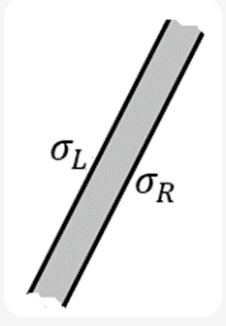Now I ask you to close your eyes. While they are closed, I move the slab parallel to the plates by an unknown amount. I then ask you to open your eyes. When you do, you see thisThen I say, "While your eyes were closed, I moved the slab parallel to the plates. Did I move it up or down and by how much?" What will you say?

This symmetry demands that Laplace's equation be one-dimensional and depend only on the horizontal variable ##x##. Given that, you can write the solution to Laplace's equation, apply the boundary conditions and voilà, the uniqueness theorem guarantees you that you have the solution for the potential everywhere. Then take derivatives and you have the electric field everywhere which is what the problem is asking for.

If you think that my symmetry argument does not apply, then you have to convince me that there is a way to figure out how far I moved the slab and in what direction without reference to the distance from the slab to the plates.

•alan123hk, Delta2 and TSny
Homework Helper
Gold Member
why with a uniform dieletric spere field changes and with slab no?
Have you thought about my questions in post #8?
What are the signs of ##\sigma_L## and ##\sigma_R##? How do their magnitudes compare?

Can you treat ##\sigma_L## and ##\sigma_R## as uniformly distributed? If so, what is their net contribution to the total electric field outside the dielectric slab?
To help decide if ##\sigma_L## and ##\sigma_R## are uniformly distributed, consider @kuruman 's suggestion that the system is invariant under translation of the slab. In particular, consider translations of the slab that are parallel to the large surfaces of the slab.

Last edited:
•Delta2 and kuruman
Homework Helper
Gold Member
why with a uniform dieletric spere field changes and with slab no?
Because Laplace's equation in the case of the sphere depends on two variables, ##r## and ##\theta## while in the slab it depends only on ##x##.

Thinking about @TSny's questions in post #8 is another way if you are not sure about the symmetry argument.

Homework Helper
Gold Member
Because Laplace's equation in the case of the sphere depends on two variables, r and θ while in the slab it depends only on x.
what breaks the symmetry in the case of the sphere, I feel like exactly the same symmetry argument as of post #15 can be used for a sphere too ...

Homework Helper
Gold Member
Only thing I can think off right now is that the normal to the surface of this slab is a constant vector, but the normal to the surface of a sphere is not constant, it depends on ##\theta,\phi##.

Homework Helper
Gold Member
Only thing I can think off right now is that the normal to the surface of this slab is a constant vector, but the normal to the surface of a sphere is not constant, it depends on ##\theta,\phi##.
I would say that there is azimuthal symmetry about the ##z##-axis which means that there are two directions that matter, one parallel to the value of unperturbed field ##\mathbf{E}_0## and one perpendicular to it. That makes Laplace's equation two-dimensional in spherical coordinates.

Yes, the normal to the surface does depend on ##\phi##, but the ##x##-axis, relative to which ##\phi## is measured, could be anywhere on the equator of the sphere. If I rotated the coordinate axes together with the normal about ##z## while your eyes were closed, you wouldn't know that I did it when you open them. Of course, I should have additional knowledge of an external coordinate system otherwise I wouldn't be able to perform the rotation and know about it.

Homework Helper
Gold Member
I would say that there is azimuthal symmetry about the ##z##-axis which means that there are two directions that matter, one parallel to the value of unperturbed field ##\mathbf{E}_0## and one perpendicular to it. That makes Laplace's equation two-dimensional in spherical coordinates.

Yes, the normal to the surface does depend on ##\phi##, but the ##x##-axis, relative to which ##\phi## is measured, could be anywhere on the equator of the sphere. If I rotated the coordinate axes together with the normal about ##z## while your eyes were closed, you wouldn't know that I did it when you open them. Of course, I should have additional knowledge of an external coordinate system otherwise I wouldn't be able to perform the rotation and know about it.
I have problems in many cases with understanding symmetry arguments and this is one case here.

But I understand it in terms of @TSny questions, in the case of a sphere the polarization surface charge density of the sphere is not canceled out exactly, therefore it has a net contribution to the field outside the sphere.

Homework Helper
Gold Member
I have problems in many cases with understanding symmetry arguments and this is one case here.

But I understand it in terms of @TSny questions, in the case of a sphere the polarization surface charge density of the sphere is not canceled out exactly, therefore it has a net contribution to the field outside the sphere.
The best to understand it is the way you can explain it to yourself.

•Delta2
alan123hk
If two infinitely large capacitor plates are infinitely far apart, and although the dielectric slab inclined in the middle is also infinitely large, but anywhere on its surface is infinitely far away from the two capacitor plates, then intuitively I believe that the symmetry argument and one-dimensional Laplace's equation should be valid.

Edit :
Imagine if we say that the electric field is curved instead of straight, how much is it curved? How far away from the dielectric slab does it get straight back? What are these curvatures calculated from?
Everything is infinite, so it seems hard to find a basis for computation. 🤔

Last edited:
•Delta2
Homework Helper
Gold Member
There are two symmetries involved here regarding the surface charge density of the slab.

The first is a symmetry that is associated with the fact that the externally applied field is uniform. For any shaped dielectric placed in the field, a translation of the dielectric will not change the charge distribution on the dielectric.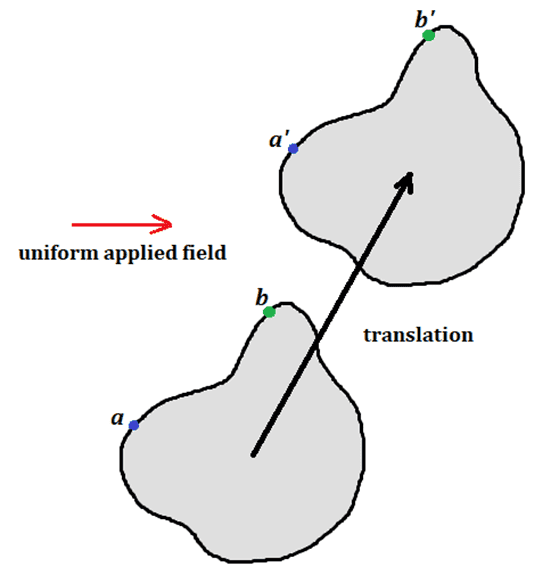So, if point ##a## gets translated to point ##a'## and ##b## to ##b'##', we can conclude that ##\sigma_a = \sigma_a'## and ##\sigma_b = \sigma_b'##. But we can't conclude anything from this symmetry about how ##\sigma_a## compares to ##\sigma_b##.

The second symmetry that we can use is that for the slab, a translation of the slab parallel to itself carries the slab into itself. Imagine the slab is placed in an arbitrary applied field that is not necessarily uniform, such as the dipole field of two fixed charges ##q## and ##-q##.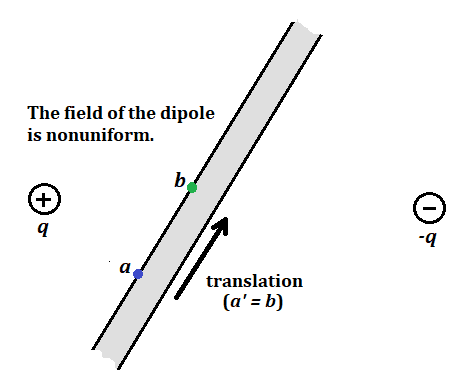Let the translation take point ##a## to point ##b##. So ##a \rightarrow a'= b## while ##b \rightarrow b'## (not shown). Point ##a'## after the translation is in the same "physical condition" as point ##b## was before the translation. So, ##\sigma_a' = \sigma_b##. But this symmetry doesn't tell us anything about how ##\sigma_a## compares to ##\sigma_b##.

For the case of the slab being in a uniform applied field, we can use both of these symmetries to deduce that ##\sigma_a = \sigma_a' = \sigma_b##. So, ##\sigma_a = \sigma_b##. For any two points ##a## and ##b## on the left face of the slab, we can construct such a translation. So, any two points on the left face have the same charge density.

Last edited:
•stefano77 and Delta2
Homework Helper
Gold Member
So @TSny in the case of a dielectric sphere in a uniform external E-field, the second symmetry doesn't work?

Homework Helper
Gold Member
So @TSny in the case of a dielectric sphere in a uniform external E-field, the second symmetry doesn't work?
That's right. For two points ##a## and ##b## on the surface of the sphere you can have a translation that takes ##a \rightarrow a' = b##. But, even if the applied field is uniform, we cannot say that the "physical condition" of point ##a'## after the translation is the same as the physical condition of point ##b## before the translation. The "physical condition" at a point on the spherical surface includes the value of the applied field at that point as well as the relation of that point to all of the other points of the sphere. For example, as you noted post #19, the orientations of the normals at ##a'## and ##b## are different.

•Delta2
stefano77
There are two symmetries involved here regarding the surface charge density of the slab.

The first is a symmetry that is associated with the fact that the externally applied field is uniform. For any shaped dielectric placed in the field, a translation of the dielectric will not change the charge distribution on the dielectric.
View attachment 302485

So, if point ##a## gets translated to point ##a'## and ##b## to ##b'##', we can conclude that ##\sigma_a = \sigma_a'## and ##\sigma_b = \sigma_b'##. But we can't conclude anything from this symmetry about how ##\sigma_a## compares to ##\sigma_b##.

The second symmetry that we can use is that for the slab, a translation of the slab parallel to itself carries the slab into itself. Imagine the slab is placed in an arbitrary applied field that is not necessarily uniform, such as the dipole field of two fixed charges ##q## and ##-q##.
View attachment 302494
Let the translation take point ##a## to point ##b##. So ##a \rightarrow a'= b## while ##b \rightarrow b'## (not shown). Point ##a'## after the translation is in the same "physical condition" as point ##b## was before the translation. So, ##\sigma_a' = \sigma_b##. But this symmetry doesn't tell us anything about how ##\sigma_a## compares to ##\sigma_b##.

For the case of the slab being in a uniform applied field, we can use both of these symmetries to deduce that ##\sigma_a = \sigma_a' = \sigma_b##. So, ##\sigma_a = \sigma_b##. For any two points ##a## and ##b## on the left face of the slab, we can construct such a translation. So, any two points on the left face have the same charge density.

stefano77
thank you so much for your observation...you have solved the problem...here's the solution...
then, ##\rho_{inside}## is null because the dieletric is homogenous and ##\sigma## is costant on all dielectric for symmetry so that we can consider the dieletric equivalent to an oblique capacitor with superficial charge as ##\sigma##...
it is cumbersome to use Laplace equatiorn in this case...I thank you everyone for the help...
l think this is the best phisics forum allover the world...
l went on physics.stackexchange before coming here, but they ignore me and give useless advice...
just one thing, may you suggest me physics forum as good as this one...thank you so much
how can l write SOLVED for this thread?

•Delta2
Homework Helper
Gold Member
thank you so much for your observation...you have solved the problem...here's the solution...
then, ##\rho_{inside}## is null because the dieletric is homogenous and ##\sigma## is costant on all dielectric for symmetry so that we can consider the dieletric equivalent to an oblique capacitor with superficial charge as ##\sigma##...
it is cumbersome to use Laplace equatiorn in this case...I thank you everyone for the help...
Yes I think you can view it as a capacitor inside another capacitor.
l think this is the best phisics forum allover the world...
l went on physics.stackexchange before coming here, but they ignore me and give useless advice...
just one thing, may you suggest me physics forum as good as this one...thank you so much
how can l write SOLVED for this thread?
Yes well, physics forums (PF) is not perfect but it is simply the best there is (in my opinion) in this planet.
Stackexchange is second by far...